Experience The Power of The Sun at its Finest.
KARACHI
LAHORE
+92 -(51)-234-8617
Solar Panel Calculator

## How Many Solar Panels Do I Need?

Our solar panel calculator makes it easy to size a solar array for your home. Answer the questions below to estimate how many solar panels you’ll need for your home, based on your location and utility consumption. Results may vary.

## Solar Panel Calculator

Use our calculator below for solar panel estimation

On average, how many KiloWatt-Hours (kWh) do you use per month? (Refer to your electric bill)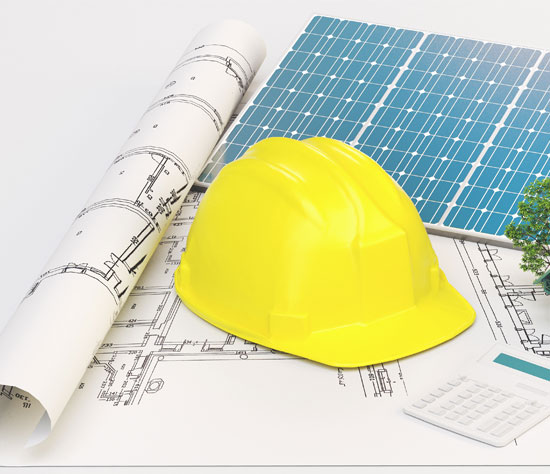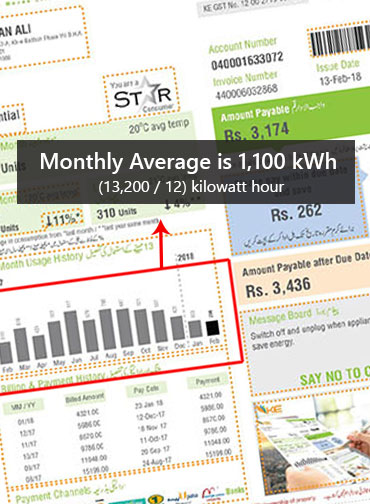01

#### Determine your average monthly electricity usage

You probably consume more electricity during certain months of the year. Refer to your electricity bills for the past 12 months, and calculate your average usage (kWh) over that period.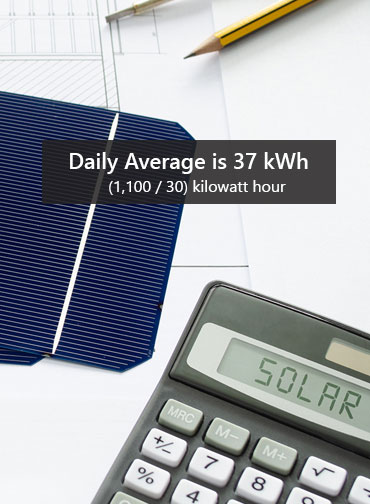02

#### Calculate your daily kWh usage

Next, you can estimate your average daily kWh usage by dividing your monthly usage by 30. For example, say your average kWh per month is 1,100. 1,100 kWh divided by 30 days (1 month) equals 37 kWh per day.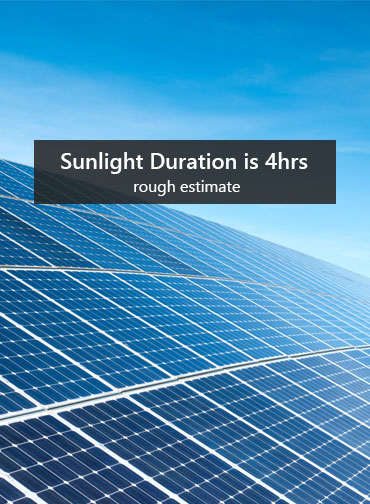03

Sunlight affects how much energy your solar panels generate. As a rough estimate, let’s assume that a 310W solar panel produces 1.24 kWh a day, and your location receives 4 hours of sunlight per day.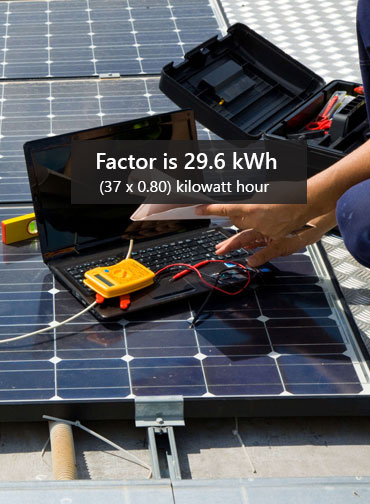04

#### Factor in your potential solar power usage

Next, how much of your current kWh usage do you want to offset with solar power? 100%? 80%? 50%? Multiply this percentage with your daily kWh usage. If you want your solar system to account for 80% of your energy consumption, here’s how the calculation would work: 37 kWh per day x 0.80 = 29.6 kWh.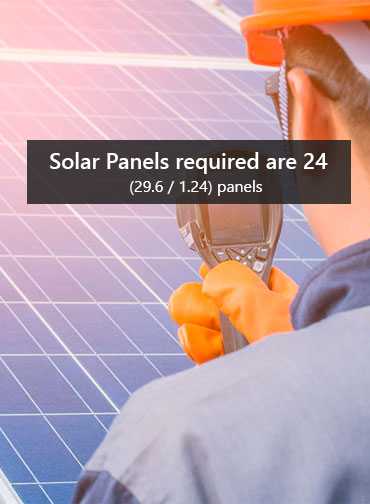05

#### Determine how many solar panels you’ll need

One solar panel produces about 1.24 kWh per day. In the example above, you would need 24 solar panels to account for 80% of your average consumption (29.6 kWh daily usage divided by 1.24 kWh per panel). Likewise, if you wanted to account for 100% of your daily kWh consumption, you would need 30 solar panels (37 kWh daily usage divided by 1.24 kWh per panel).
Get in touch